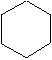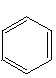`Chem 122 Laboratory, Spring 1996      Name________________________________`

## Molecular Modeling with "ball-and-stick" model kits

For this experiment you will work in pairs. Pick a partner and unpack your model kit.

1. Why do you think the different types of atoms are given different colors? Are these colors the real colors of these atoms, or just arbitrary colors?

```
```
2. Locate on the periodic table in your textbook all the atom types included in the kit.

3. How many holes (for bonds) are there in these atoms?

```                H            hydrogen               _____
C            carbon                 _____
O            oxygen                 _____
Cl           chlorine               _____
Br           bromine                _____```
4. Construct models for the diatomic molecules hydrogen (H2), chlorine (Cl2), and hydrogen chloride (HCl). What does the bond between the two atoms represent; that is, what is the bond actually composed of?

```
```
5. What is the "octet rule" and how does it explain the tendency of oxygen to form two bonds, whereas chlorine, for example, forms only one bond?

```
```
6. Construct models for methane (CH4) and for chloroform (CHCl3). What shape is formed by the vertices of the molecule?

```
```
7. Construct models for ethane (C2H6), and propane (C3H8). Is there any other way to construct these molecules?

```
```
8. Construct a model for butane (C4H10). There is more than one way to construct a molecule with the formula C4H10. How many can you find? Sketch their structures.

```
```
9. Ethanol and methyl ether both have the empirical formula C2H6O but different structures. Ethanol is an alcohol and has an -OH group. Ether has a -O- linkage. Construct both molecules and draw their structures here:

```
```
10. Some molecules contain so-called "double bonds". In the modeling kit, the curved bonds are used used allow double bonds between atoms. Build models for diatomic oxygen and for carbon dioxide. How many double bonds do these molecules have?

```

```
11. Octane (found in gasoline) has 8 carbons and is one of the family of hydrocarbons such as methane, ethane, butane, and propane. What formula would you predict for octane? Can you some up with a general expression that should work for all hydrocarbons of this type? E.g. CnHm, given n, what is m?

```
```
12. Chains and rings.

Many organic molecules have chains of carbon atoms. It is also common for atoms to form closed rings. Most drugs, for example, contain rings or 5 or 6 atoms.

Using your molecular model kit, construct a hydrocarbon with the formula C6H12, consisting of a ring of six CH2 groups. This is called cyclohexane. Then construct another hydrocarbon with the formula C6H6, consisting of a ring of six CH groups with alternating single and double bonds between the carbons. This is called benzene, and it is by far the most common ring structure, found over and over again in many different naturally occurring organic compounds. The short-hand line-segment representations of these two structures are:cyclohexane benzene

a. What geometrical arrangement is characteristic of the atoms in the benzene molecule but not those of the cyclohexane molecule?

```
```
b. What is the smallest ring of CH2 units you have make without straining the bonds?

```
```
c. Many years ago chemists tried to create a molecule called cubane that would consist of a cube of 8 carbon atoms, with two hydrogens attached to each carbon. This proved not to be stable enough to isolate. Attempt to build a model of cubane and explain why the molecule is not stable.

```
```
13. Chiral (left- and right-handed) molecules.

a. Construct a model of a molecule that has a single carbon atom in the center and four different atoms or groups attached to it. Construct its mirror image also. Are the two mirror pairs really identical or are they distinct? Hint: try to superimpose them by laying them side by side.

```
```
b. Place the model against a piece of paper so that three of the atoms touch the paper. Mark the positions of each touching atom on the paper. Then try to match the same three positions with the other mirror image. Do both fit, or only one?

```
```
c. Construct a model that has a carbon atom in the center but only three different atoms attached to it, that is, that has two of one kind of atom instead of four different atoms. Make its mirror image also. Are the two mirror pairs really identical or are they distinct?

```
```
d. Try part b with this model. Do both fit or only one?

e. What would you predict for carbons that have fewer than three different attached atoms? Would this give distinct left and right handed forms or not? On the basis of these observations, formulate a rule for predicting when a carbon atom will give distinct left and right handed forms.

```

```
f. Construct a model of lactic acid (Atkins, page 48). How could you demonstrate whether or not this molecule is chiral (has distinct left and right-handed forms)? Does one of the carbons have four different groups attached to it? Which one?

```

```
i. How could you predict that carvone (Atkins, page 133) would be chiral, on the basis of its structure, without actually building a model the molecule?

```

```
What observable property of the two chiral forms of carvone is different?

```

```
g. Construct models of the left- and right-handed forms of thalodimide (Atkins, page 174). What important difference between these forms lead to the thalidomide tragedy in the 50's.

Maryland Collaborative for Teacher Preparation